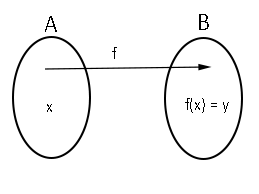# Function of One Variable

By Suresh Chand

The goal of study function of one variable is after understanding functions. In this unit functions can be represent by different ways.

### Function of One Variable

A function of one variable x is a prescription y(x), which calculates a number, the function value, for any feasible value of the variable x.

Let A and B be the non-empty sets. then a rule A to B is called function if every element of domain A has unique association with elements of co-domain B.

Let $$f:A \rightarrow B$$ be a function

1. Set A is called function
2. Set B is called co-domain
3. y is called image of x under  i.e. y = f(x)
4. x is called pre-image of y
5. Range oof f is denoted by f(A) and defined by f(A) = $$\left \{ f(x) : \forall \enspace x \enspace \varepsilon \enspace A \enspace \right \}$$## Identify Domain and Range

### i) y = x2

Solution:

Given y = x2

For domain, for all real values of x, y exists. So, domain is set of all real number i.e. domain is (-∞, ∞)

For Range,  y = x2  or $$x = \sqrt{y}$$ . For all $$y \geq 0$$, x is defined. Thus, y is set of all non-negative and real number. Hence, range is $$y \geq 0$$ or [0, ∞).

### ii) $$y = \frac{1}{x}$$

Solution:

For Domain, when x = 0, y doesn’t exist, it means y is exists for all real number except 0. The domain is set of all real number except 0 i.e. (-∞, 0) U (0, ∞)

For Range, $$y = \frac{1}{x}$$ or $$x = \frac{1}{y}$$. Here x is exists for all real number except y = 0. Thus, the range is set of all real number except o i.e. (-∞, 0) U (0, ∞)

### iii) $$y = \sqrt{9-x^{2}}$$

Solution:

For Domain:

y is exists for $$9-x^{2} \geq 0$$

i.e. $$x^{2} – 9 \geq 0$$

i.e. $$(x-3)(x+3) \leq 0$$

i.e. $$-3 \leq x \leq 3$$

Hence, domain is $$-3 \leq x \leq 3$$ i.e. [-3, 3]

For Range:

$$y = \sqrt{9 – x^2}$$ $$\Rightarrow y ^ 2 = 9 – x ^ 2$$ $$\Rightarrow x = \sqrt{9 – y^2}$$

Here, x exists for $$9-y^{2} \geq 0$$

i.e. $$y^{2} – 9 \geq 0$$

i.e. $$(y-3)(y+3) \leq 0$$

i.e. $$-3 \leq y \leq 3$$

But given $$y = \sqrt{9-x^{2}}$$, which is non-negative. hence range is $$0 \leq y\leq 3$$ i.e. [0, 3]

## Even and Odd Function: Symmetry

A function y = f(x) is even function if f(-x) = f(x), and odd function if f(-x) = -f(x) for every value of x.

Note that the graph of even function is symmetrical about y-axis and the graph of odd function is symmetrical about origin.

### Identify the Symmetry

#### $$f(x) = \frac{1}{x^{-4}}$$

Solution:

Since,  $$f(x) = x^{-4} = \frac{1}{x^{4}}$$

So, $$f(-x) = \frac{1}{(-x)^{4}} = \frac{1}{x^4} = f(x)$$

Therefore, the given $$f(x) = x^{-4}$$ is even function.

## Combination of Functions

Like numbers function can be added, subtracted, multiplied and divided to product new function. Let f an g are two functions then f + g, f – g, f.g and $$\frac{f}{g}$$ are new function which are defined by

Sum: (f + g)(x) = f(x) + g(x)

Difference: (f – g)(x) = f(x) – g(x)

Product: (f.g)(x) = f(x) . g(x)

Quotient: ($$\frac{f}{g}$$)(x) = $$\frac{f(x)}{g(x)}$$

### Composite Functions

Let f and g are functions, then composite function fog is defined by

(fog)(x) = f(g(x))

Note that the domain of fog(x) is intersection of domain of g(x) and f(g(x)).

Example: If $$f(x) = \sqrt{x}$$ and [M]$$g(x) = \sqrt{2-x}$$

Find the each function and its domain

a) fog   b) gof    c) fof     d) gog

Solution:

(a)        $$fog(x) = f(g(x)) = f(\sqrt{2-x})$$

$$\sqrt{\sqrt{2-x}} = (2-x)^{\frac{1}{4}}$$

To find domain of fog

Fist we find domain of $$g(x) = \sqrt{2-x}$$

Domain of g(x) is

$$2 – x \geq 0$$ $$x \leq 2$$

Here, (-∞, 2] is domain of fog.

(b)        $$gof(x) = f(f(x)) = f(\sqrt{x}) = \sqrt{2 – \sqrt{x}}$$

To Find the domain of gof, Follow steps of question (a)

Domain of gof = [0, 4]

(c)         $$f.f(x) = f(f(x)) = f(\sqrt{x})$$

$$= \sqrt{\sqrt{x}} = x^{\frac{1}{4}}$$

To Find the domain of fof, Follow steps of question (a)

Domain of fof = [0, ∞]

(d)          $$g.g(x) = g(g(x)) = g(\sqrt{2 – x}) = \sqrt{2 – \sqrt{2 – x}}$$

To Find the domain of gog, Follow steps of question (a)

Domain of fof = [-2, 2]

## Inverse of Function

Let f be a one to one function with domain A and range B. Then its inverse function f-1 has domain B and range A and is defined by

$$f^{-1}(y) = x \Leftrightarrow f(x) = y$$

Example: Find inverse of f(x) = x3 + 2

Solution:

Since y = x3  + 2

or   x3 = y – 2

or  $$x = (y – 2)^{\frac{1}{3}}$$

or    $$f^{-1}(y) = (y – 2)^{\frac{1}{3}}$$

or    $$f^{-1}(x) = (x – 2)^{\frac{1}{3}}$$ is the required formula for inverse function.

The domain of f is range of f-1 and range of domain of f-1 and the graph of f-1 is obtained by reflecting the graph of f about line y = x

Important Questions